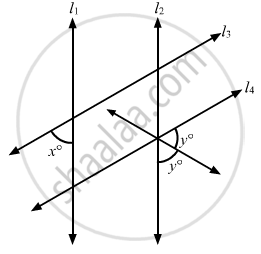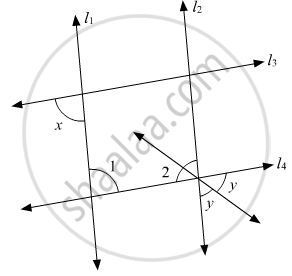# In the Given Figure, If L1 || L2 and L3 || L4, What is Y in Terms of X? - Mathematics

MCQ

In the given figure, if l1 || l2 and l3 || l4, what is y in terms of x?#### Options

•  90 + x

•  90 + 2x

•  90 + x/2

• 90 − 2x

#### Solution

The given figure is:Here, we have ∠2 and 2y are vertically opposite angles. Therefore,

∠2 = 2y  ...(i)

∠1 and x are alternate interior opposite angles.

Thus,

∠1 = x     ...(ii)

∠1 and ∠2 are consecutive interior angles.

Theorem states: If a transversal intersects two parallel lines, then each pair of consecutive interior angles are supplementary.

Thus,

∠1 + ∠2 180°

From (i) and (ii), we get:

x +2y = 180°

2y = 180° - x

y = (180° -x)/2

y = (180°)/2 - x /2

y = 90° - x /2

Concept: Parallel Lines and a Transversal
Is there an error in this question or solution?

#### APPEARS IN

RD Sharma Mathematics for Class 9
Chapter 10 Lines and Angles
Q 17 | Page 54